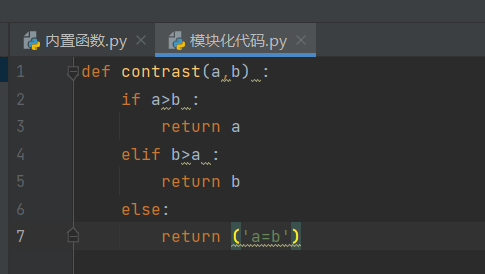# Python自定义函数

### 一、自定义函数的固定语句

```def contrast(a,b) :      #使用def来定义一个名称为contrast的方法,a与b的值是两个变量，称为形参
if a>b :       #使用条件语句进行判定
return a           #返回a的值
elif b>a :
return b           #返回b的值
else:
return ('a=b')    #返回字符串a=b

c = contrast(1,1)     #实例化方法contrast
print(c)           #输出c```

a=b

```import random       #引用随机函数
x = random.randint(1,5)    #使用随机函数中的随机整数方法
y = random.randint(1,5)
c = contrast(x,y)         #调用contrast方法，这时变量x和y,称为实参
print(x,y,c)```

1 1 a=b

### 二、模块化自定义代码的引用```import random
from 模块化代码 import *      #从模块化代码文件中引用所有方法，引用指定方法就写方法名称就可以
x = random.randint(1,5)
y = random.randint(1,5)
c = contrast(x,y)
print(x,y,c)```

2 5 5

### 三、可变变量和不可变变量

```def f(a) :       #定义方法f()
a = 'boy'
return a

b = 'girl'
c = f(b)         #实例化方法f()
print(c)```

boy

```def f(a) :          #定义方法f()
a.append('boy')     #追加字符串
return a

b = ['girl']
c = f(b)    #实例化方法f()
print(c)```

`['girl', 'boy']     #列表是可变的，使用追加方法。`

### 四、自定义函数方法中的避免被另一个文件调用的语句

```def contrast(a,b) :
if a>b :
return a
elif b>a :
return b
else:
return ('a=b')
if __name__ == '__main__' :  #写在这个语句下的所有代码都不会被调用，只在本文件中运行
def f(a):
a.append('boy')
return a
b = ['girl']
c = f(b)
print(c)```